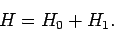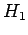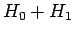Next: Improved Notation Up: Time-Independent Perturbation Theory Previous: Time-Independent Perturbation Theory

# Introduction

Consider the following very commonly occurring problem. The Hamiltonian of a quantum mechanical system is written(854)

Here,is a simple Hamiltonian whose eigenvalues and eigenstates are known exactly.introduces some interesting additional physics into the problem, but is sufficiently complicated that when we add it towe can no longer find the exact energy eigenvalues and eigenstates. However,can, in some sense (which we shall specify more precisely later on), be regarded as being small compared to. Can we find approximate eigenvalues and eigenstates of the modified Hamiltonian,, by performing some sort of perturbation expansion about the eigenvalues and eigenstates of the original Hamiltonian,? Let us investigate.

Incidentally, in this chapter, we shall only discuss so-called time-independent perturbation theory, in which the modification to the Hamiltonian,, has no explicit dependence on time. It is also assumed that the unperturbed Hamiltonian,, is time-independent.

Richard Fitzpatrick 2010-07-20